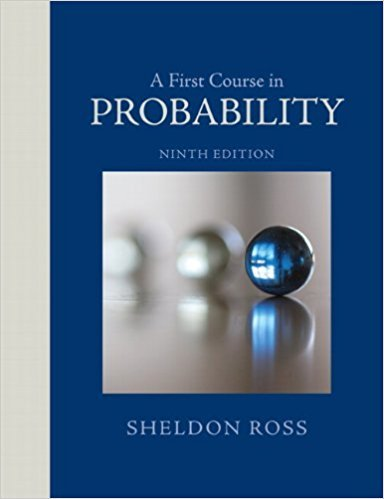×
Get Full Access to A First Course In Probability - 9 Edition - Chapter 4 - Problem 59p
Get Full Access to A First Course In Probability - 9 Edition - Chapter 4 - Problem 59p

×

# If you buy a lottery ticket in 50 lotteries, in each ofISBN: 9780321794772 63

## Solution for problem 59P Chapter 4

A First Course in Probability | 9th Edition

• Textbook Solutions
• 2901 Step-by-step solutions solved by professors and subject experts
• Get 24/7 help from StudySoup virtual teaching assistantsA First Course in Probability | 9th Edition

4 5 1 284 Reviews
11
3
Problem 59P

Problem 59P

If you buy a lottery ticket in 50 lotteries, in each of which your chance of winning a prizeis what is the (approximate) probability that you will win a prize

(a) at least once?

(b) exactly once?

(c) at least twice?

Step-by-Step Solution:

Solution:

Step 1 of 4:

We have the number of trials n = 50.

The probability of winning a prize is p =.

Let X follows the Poisson distribution with the probability mass function

P(X = x) =Where= np

= 50()

= 0.5.

Step 2 of 4

Step 3 of 4

##### ISBN: 9780321794772

Unlock Textbook Solution